• 一、学习心得： 在我学习基本滤波算法原理的时候，因为刚接触不是很理解算法具体是怎样实现的，不过在学习了图像形态学之后，发现滤波算法其实很简单。所以在此建议初学者在...而在图像处理中的滤波算法中，处理的
一、学习心得：
在我学习基本滤波算法原理的时候，因为刚接触不是很理解算法具体是怎样实现的，不过在学习了图像形态学之后，发现滤波算法其实很简单。所以在此建议初学者在学习滤波算法之前，可以先学习一下图像形态学，会达到事半功倍的效果。

二、对于滤波功能的理解：
滤波算法，可以理解成一种过滤算法，就像我们筛选产品时，把次品去除掉，只留下合格的产品。而在图像处理中的滤波算法中，处理的对象是图像，除了去除掉图像中不想要的像素点的值（如去除噪声），还可以加强图像中我们需要研究一些内容（如边缘提取）。

三、滤波算法：
这里所讲的算法都是针对图像空间的滤波算法，其中模板，可以理解为图像形态学中的结构元素，是用来选取图像中的那些像素点被用来操作的。空间滤波根据其功能划分为平滑滤波和锐化滤波。平滑滤波：能减弱或者消除图像中高频率分量，但不影响低频率分量，在实际应用中可用来消除噪声。锐化滤波：与平滑滤波相反，能减弱或者消除图像中低频率分量，但不影响高频率分量，可使图像反差增加，边缘明显。实际应用可用于增强被模糊的细节或者目标的边缘。

空间增强滤波技术分类：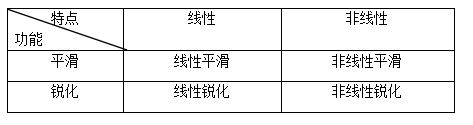1、线性平滑滤波

（1）方框滤波：用一个像素的领域像素值之和作为滤波结果，邻域即模板所覆盖的图像区域，此时模板的所有系数都为1.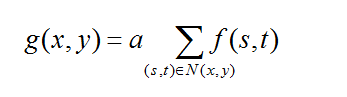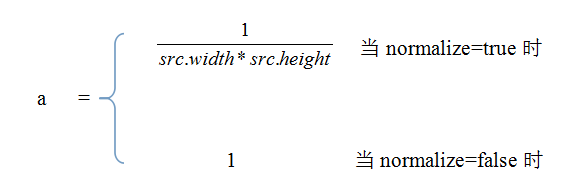（2）邻域平均：是特殊大方框滤波，用一个像素的领域平均像素值作为滤波结果，即a为第一种情。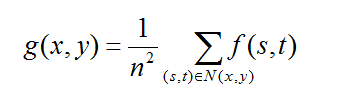其中N(x,y)为模板岁覆盖的图像的区域，n为模板的尺寸。

（3）加权平均：此时的模板系数不是1，而是具体的系数。一般认为距离模板中心的像素应对滤波结果有较大的贡献，所以可将接近模板中心的系数取得比模板周边的系数。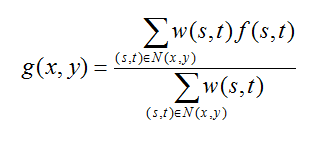（4）高斯平均：是一种特殊的加权平均，只不过模板中的系数由高斯分布来确定的。

2、线性锐化滤波

（1）拉普拉斯算子
拉普拉斯算子是一种各向同性的二阶微分算子，利用微分系数来确定模板系数，然后再与图像进行卷积运算，从而实现锐化滤波。

根据拉普拉斯定义：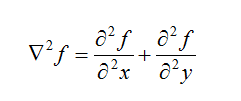两个分别沿X和Y方向的二阶偏导均可借助差分计算：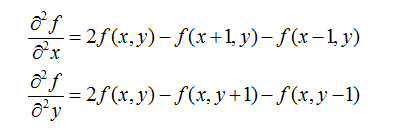合并为：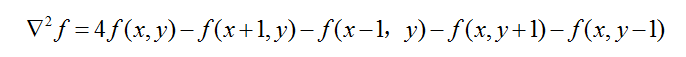当模板为4-邻域时                          当模板为8-邻域时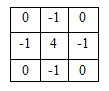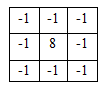以上两种模板的系数之和为0，这是为了使经过模板运算的图像的均值不变。拉普拉斯算子增强了图像中的灰度不连续区域，而减弱了图像中灰度值缓慢变化区域对比度，将这样的结果叠加到原始图像中，就可以得到瑞华后的额图像。


（2）高频提升滤波
图像的锐化效果可以通过叠加图像的微分结果得到，也可以通过减除图像积分结果得到。
设原始图像为f(x,y),平滑后的图像为g(x,y)：

非锐化掩模：h(x,y) = f(x,y)-g(x,y)

锐化图像：{ f(x,y)- g(x,y) } + f(x,y）

高频提升滤波：把院士图形乘以一个放大系数A，再减去平滑图像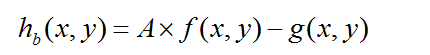可转化为：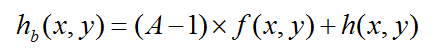当A=1时，为非锐化掩模；
当A=2时，为非锐化掩模化。

3、非线性平滑滤波

（1）中值滤波：对模板下对应的像素值进行升序排序，选取中间值作为结果。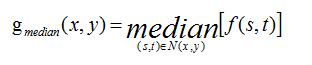（2）与中值滤波类似的，还有最大值、最小值、中点滤波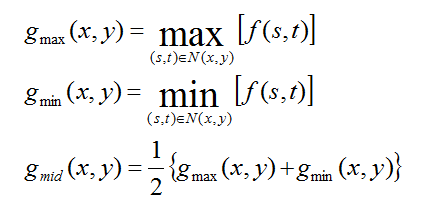以上四种滤波也称之为百分比滤波，百分比滤波基于模板的排序来工作，又叫作序统计滤波。
展开全文图像处理 滤波 图像增强
• 该篇主要是对图像滤波算法一个整理，主要参考的大神的博客： https://blog.csdn.net/qq_15606489/article/details/52755444 1：图像滤波既可以在实域进行，也可以在频域进行。图像滤波可以更改或者增强图像。通过...
该篇主要是对图像滤波算法一个整理，主要参考的大神的博客：
https://blog.csdn.net/qq_15606489/article/details/52755444
1：图像滤波既可以在实域进行，也可以在频域进行。图像滤波可以更改或者增强图像。通过滤波，可以强调一些特征或者去除图像中一些不需要的部分。滤波是一个邻域操作算子，利用给定像素周围的像素的值决定此像素的最终的输出值。
图像滤波可以通过公式：
O(i,j)=∑m,nI(i+m,j+n)∗K(m,n)$O\left(i,j\right)=\sum _{m,n}^{}I\left(i+m,j+n\right)\ast K\left(m,n\right)$$O(i,j) = \sum_{m,n}^{ }I(i+m,j+n)*K(m,n)$
其中K为滤波器，在很多文献中也称之为核（kernel）。常见的应用包括去噪、图像增强、检测边缘、检测角点、模板匹配等。

2：均值滤波
用其像素点周围像素的平均值代替元像素值，在滤除噪声的同时也会滤掉图像的边缘信息。在OpenCV中，可以使用boxFilter和blur函数进行均值滤波。均值滤波的核为：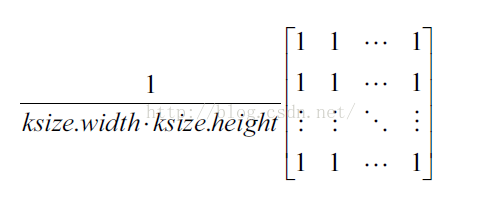3：中值滤波
中值滤波用测试像素周围邻域像素集中的中值代替原像素。中值滤波去除椒盐噪声和斑块噪声时，效果非常明显。在OpenCV中，可以使用函数medianBlur进行操作。

4：高斯滤波
这里参考一位大神的博客写的很细很好明白：https://blog.csdn.net/nima1994/article/details/79776802
总结一下：

像均值滤波，是简单的取平均值，模板系数都是1。而图像上的像素实际上是坐标离散但是值却连续的，因为越靠近的点关系越密切，越远离的点关系越疏远。因此，加权平均更合理，距离越近的点权重越大，距离越远的点权重越小。
既然是依据距离来加权平均，那么很容易想到高斯函数f(x)=1σ2π√e−(x−μ)22σ2$f\left(x\right)=\frac{1}{\sigma \sqrt{2\pi }}{e}^{\frac{-\left(x-\mu {\right)}^{2}}{2{\sigma }^{2}}}$$f(x) = \frac{1}{\sigma\sqrt{2\pi }}{e}^{\frac{-(x-\mu )^{2}}{2\sigma^2}}$，如图所示：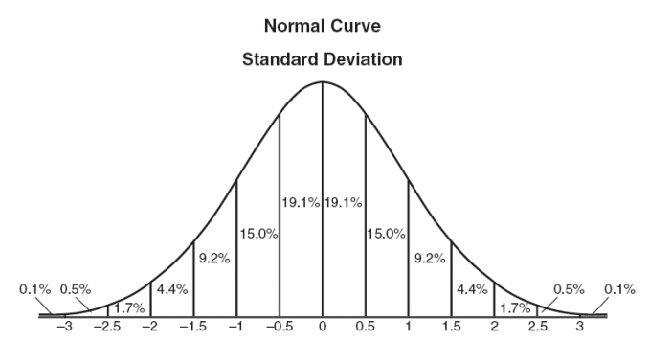从高斯函数来看，离原点距离越近，得到的权重越高，越远离原点，得到的权重越小。
上边是一维的高斯函数，当中心点为原地时，x$x$$x$的均值μ=0$\mu =0$$\mu = 0$，此时f(x)=1σ2π√e−x22σ2$f\left(x\right)=\frac{1}{\sigma \sqrt{2\pi }}{e}^{\frac{-{x}^{2}}{2{\sigma }^{2}}}$$f(x) = \frac{1}{\sigma\sqrt{2\pi }}{e}^{\frac{-x^{2}}{2\sigma^2}}$
由于图像是二维矩阵，则采用二维高斯函数f(x,y)=12πσ2e−(x2+y2)2σ2$f\left(x,y\right)=\frac{1}{2\pi {\sigma }^{2}}{e}^{\frac{-\left({x}^{2}+{y}^{2}\right)}{2{\sigma }^{2}}}$$f(x,y) = \frac{1}{{2\pi }\sigma^2}{e}^{\frac{-(x^2+y^2 )}{2\sigma^2}}$有了这个函数就可以计算滤波模板中每个点的权重了。
权重矩阵：
假定中心点是（0，0），那么距离它最近的8个点的坐标如下：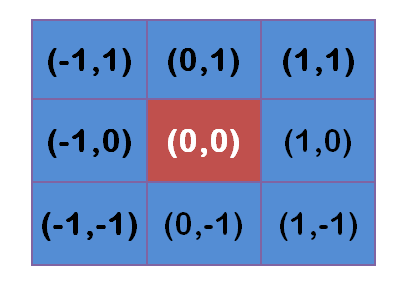更远的点以此类推。
将这模板中的坐标x,y代入到二维高斯函数中，假定μ=0$\mu =0$$\mu = 0$，σ$\sigma$$\sigma$为1.5,则模糊半径为1的权重矩阵如下: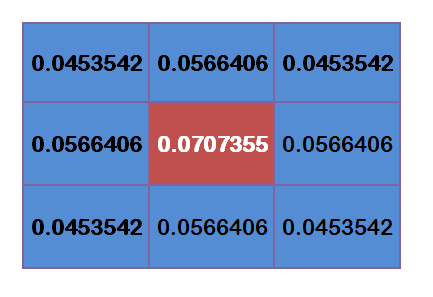这9个点的权重总和等于0.4787147，如果只计算这9个点的加权平均，还必须让它们的权重之和等于1，因此上面9个值还要分别除以0.4787147，得到最终的权重矩阵。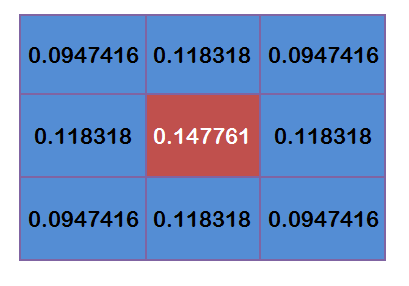计算高斯滤波的结果
假设现在3*3高斯滤波器覆盖的图像的像素灰度值为：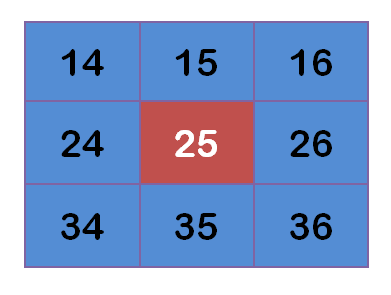每个点与上边计算得到的9个权重值相乘：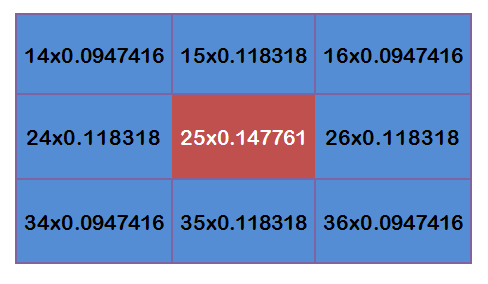得到：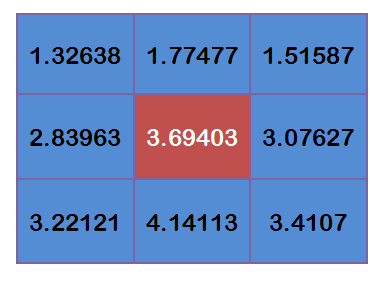将这9个值加起来，就是中心点的高斯模糊的值。

对所有点重复这个过程，就得到了高斯模糊后的图像。如果原图是彩色图片，可以对RGB三个通道分别做高斯模糊。

边界处理
如果一个点处于边界，周边没有足够的点，怎么办？

一个变通方法，就是把已有的点拷贝到另一面的对应位置，模拟出完整的矩阵。

5：双边滤波
参考了大神的博客：http://www.360doc.com/content/17/0306/14/28838452_634420847.shtml
高斯滤波只考虑了周边点与中心点的空间距离来计算得到权重。首先，对于图像滤波来说，一个通常的intuition是：（自然）图像在空间中变化缓慢，因此相邻的像素点会更相近。但是这个假设在图像的边缘处变得不成立。如果在边缘处也用这种思路来进行滤波的话，即认为相邻相近，则得到的结果必然会模糊掉边缘，这是不合理的，因此考虑再利用像素点的值的大小进行补充，因为边缘两侧的点的像素值差别很大，因此会使得其加权的时候权重具有很大的差别。可以理解成先根据像素值对要用来进行滤波的邻域做一个分割或分类，再给该点所属的类别相对较高的权重，然后进行邻域加权求和，得到最终结果。
双边滤波与高斯滤波相比，对于图像的边缘信息能够更好的保留，其原理为一个与空间距离相关的高斯核函数与一个灰度距离相关的高斯函数相乘。
空间距离：e−(xi−xc)2+(yi−yc)22σ2${e}^{-\frac{\left({x}_{i}-{x}_{c}{\right)}^{2}+\left({y}_{i}-{y}_{c}{\right)}^{2}}{2{\sigma }^{2}}}$$e^{-\frac{(x_i - x_c)^2 + (y_i - y_c)^2}{2\sigma^2}}$，其中(xc,yc)$\left({x}_{c},{y}_{c}\right)$$(x_c,y_c)$是中心点坐标，比如为(0,0)，(xi,yi)$\left({x}_{i},{y}_{i}\right)$$(x_i,y_i)$为当前点的坐标，σ$\sigma$$\sigma$为空间域标准差。
灰度距离：e−(gray(xi,yi)−gray(xc,yc))22σ2${e}^{-\frac{\left(gray\left({x}_{i},{y}_{i}\right)-gray\left({x}_{c},{y}_{c}\right){\right)}^{2}}{2{\sigma }^{2}}}$$e^{-\frac{(gray(x_i,y_i) - gray(x_c,y_c))^2}{2\sigma^2}}$，其中gray(xi,yi)$gray\left({x}_{i},{y}_{i}\right)$$gray(x_i,y_i)$是当前像素点的灰度值，gray(xc,yc)$gray\left({x}_{c},{y}_{c}\right)$$gray(x_c,y_c)$是模板中覆盖图片区域的中心点像素的灰度值，也就是(0,0)处的灰度值，σ$\sigma$$\sigma$为值域标准差。
对于高斯滤波，仅用空间距离的权值系数核与图像卷积后确定中心点的灰度值。即认为离中心点越近，其权值系数越大。
双边滤波中加入了对灰度信息的权重，即在领域内，灰度值越接近中心点灰度值的点的权值更大，灰度值相差大的点权重越小。其权重大小则由值域高斯函数确定。
两者权重系数相乘，得到最终的卷积模板，由于双边滤波需要每个中心点领域的灰度信息来确定其系数，所以速度比一般的滤波慢得多，而且计算量增长速度为核的大小的平方。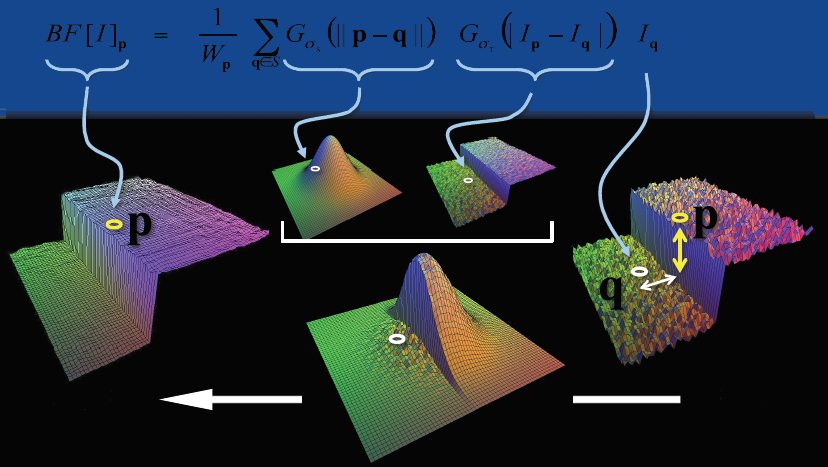参数的选择：空间域σ$\sigma$$\sigma$的选取，和值域σ$\sigma$$\sigma$的选取。
结论：σ$\sigma$$\sigma$越大，边缘越模糊；σ$\sigma$$\sigma$越小，边缘越清晰。
展开全文图像处理
• 图像处理中有两篇滤波算法论文我认为是比较实用且有新颖性，今天就在这里将论文的主要思想介绍一下，这两种算法非常容易与其他算法结合，更主要是学习里面蕴含的思想。 注意：线性滤波器的线性组合仍是线性滤波器。...
图像处理中有两篇滤波算法论文我认为是比较实用且有新颖性，今天就在这里将论文的主要思想介绍一下，这两种算法非常容易与其他算法结合，更主要是学习里面蕴含的思想。
注意：线性滤波器的线性组合仍是线性滤波器。

1. Guided Filter

一开始看到有Guided 这个词，就容易想到，它是由外部的某些已知条件来引导滤波器发挥作用达到滤波的效果。其实，这是一个非常直观的操作：希望参考图像能够给我们提供某种信息来得到一个结果，比如说利用特征信息来保留原有的特征而尽量平滑原图。He Kaiming等人把这种很直接的认识转化为了数学语言，并用简单的方法实现，也是该论文工作对我最大的启发–工作要越简单越有效。
由此引出，设引导图为I，原图为p，输出图为q，Guided filter的表达式为：

qi=akIi+bk,  i∈window pixels(1)q_i=a_kI_i+b_k,\ \ i\in window \ pixels\qquad(1)

对于一个滤波器来说，邻域信息怎么利用是最重要的，本文利用图像内窗口的局部线性模型来确保输出q跟I有类似的边缘信息，即用aI+b$aI+b$来拟合回归得到q，所以要求解系数ak,bk$a_k,b_k$，采用这样的一个代价函数：

对代价函数采用最小二乘法来估计ak,bk$a_k,b_k$。

参考下图帮助理解：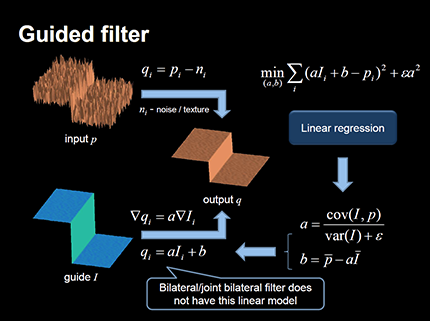输出就直接参考公式（1）得到q，所以它是能做到保边模糊的效果的。

2. Weighted Least Square Filter

当然加权最小平方滤波器是在中最早出现，它的目的是找到能够在梯度小的区域尽量平滑，梯度比较大的区域（边缘区）尽量跟原图保持一致。因此，可以建立这样的一个目标函数：

其实，它就可以当作是min (u−g)2+λb$min\ (u-g)^2+\lambda b$，后面项是约束项，用来控制平滑的程度。
重写（5）式：

对（6）求导，可以求u最小：

中还有很多具体的细节，感兴趣的可以去看论文中的叙述，这篇论文有一定的干货。GF和WLS两者其实是有很大相似之处的，原理都不复杂，但是效果出众。
这里还有个很有意思的信号拟合图，可以看出各种方法对信号平滑区与变化区处理的不同：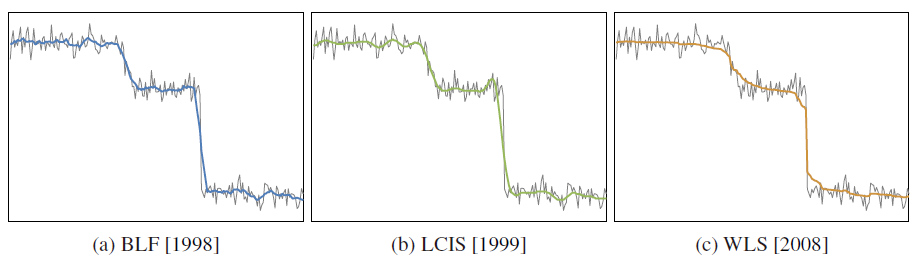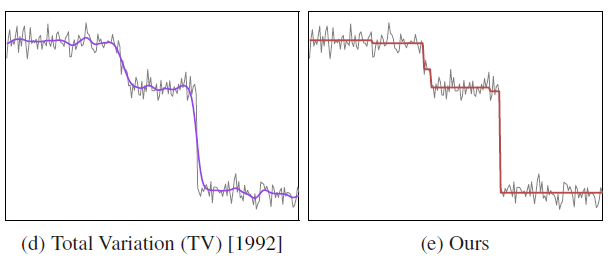ours是指中提出的方法，如果在遇到对信号做近似或重建处理时，可以根据目的来选择其中的一种方法，这里只是提供了形象的示意图，具体的可能需要考虑更多的内容来进行方法和约束项的选择。

 He, Kaiming. Sun, Jian. Tang, Xiaoou. Guided Image Filtering. European Confenrence on Computer Vision. 2010, 7.
 Farbman, Zeev. Fattal, Raanan. Lischinski, Dani. Szeliski, Richard. Edge-preserving decompositions for multi-scale tone and detail manipulation. ACM Transactions on Graphics. 2008(27), 3.
 LAGENDIJK, R. L., BIEMOND, J., AND BOEKEE, D. E. 1988.Regularized iterative image restoration with ringing reduction. IEEE Trans. Acoustics, Speech, and Signal Proc., Speech, Signal Proc. 36, 12 (December), 1874–1888.
Li Xu,Cewu Lu, Yi Xu, Jiaya Jia. Image Smoothing via L0 Gradient Minimization. ACM TOG. 2011, 30, 6.

展开全文filter 图像处理 算法
• 转自：openCV之滤波&amp;均值滤波（及代码实现）：https://blog.csdn.net/weixin_37720172/article/details/72627543  在开始我们今天的博客之前，我们需要先了解一下什么是滤波： openCV之滤波&...
转自：openCV之中值滤波&均值滤波（及代码实现）：https://blog.csdn.net/weixin_37720172/article/details/72627543

在开始我们今天的博客之前，我们需要先了解一下什么是滤波：

openCV之中值滤波&均值滤波（及代码实现）首先我们看一下图像滤波的概念。图像滤波，即在尽量保留图像细节特征的条件下对目标图像的噪声进行抑制，是图像预处理中不可缺少的操作，其处理效果的好坏将直接影响到后续图像处理和分析的有效性和可靠性。

下图左边是原图右边是噪声图：消除图像中的噪声成分叫作图像的平滑化或滤波操作。信号或图像的能量大部分集中在幅度谱的低频和中频段是很常见的，而在较高频段，感兴趣的信息经常被噪声淹没。因此一个能降低高频成分幅度的滤波器就能够减弱噪声的影响。
图像滤波的目的有两个:一是抽出对象的特征作为图像识别的特征模式;另一个是为适应图像处理的要求，消除图像数字化时所混入的噪声。
而对滤波处理的要求也有两条:一是不能损坏图像的轮廓及边缘等重要信息;二是使图像清晰视觉效果好。
平滑滤波是低频增强的空间域滤波技术。它的目的有两类：一类是模糊；另一类是消除噪音。
空间域的平滑滤波一般采用简单平均法进行，就是求邻近像元点的平均亮度值。邻域的大小与平滑的效果直接相关，邻域越大平滑的效果越好，但邻域过大，平滑会使边缘信息损失的越大，从而使输出的图像变得模糊，因此需合理选择邻域的大小。
关于滤波器，一种形象的比喻法是：我们可以把滤波器想象成一个包含加权系数的窗口，当使用这个滤波器平滑处理图像时，就把这个窗口放到图像之上，透过这个窗口来看我们得到的图像。

举一个滤波在我们生活中的应用：美颜的磨皮功能。如果将我们脸上坑坑洼洼比作是噪声的话，那么滤波算法就是来取出这些噪声，使我们自拍的皮肤看起来很光滑。

这篇博文会介绍中值滤波以及均值滤波两种算法

一.均值滤波

图片中一个方块区域（一般为3*3）内，中心点的像素为全部点像素值的平均值。均值滤波就是对于整张图片进行以上操作。

我们可以看下图的矩阵进行理解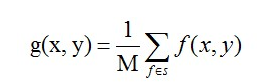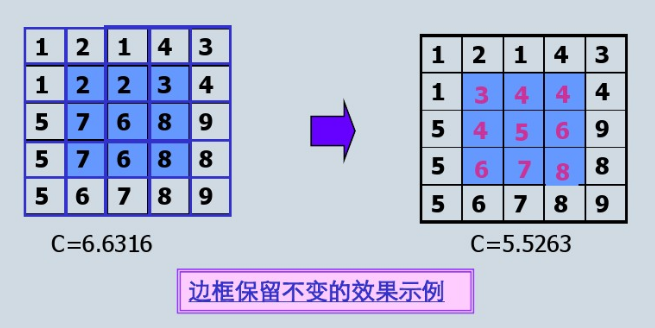缺陷：均值滤波本身存在着固有的缺陷，即它不能很好地保护图像细节，在图像去噪的同时也破坏了图像的细节部分，从而使图像变得模糊，不能很好地去除噪声点。特别是椒盐噪声

实现代码：

#include "opencv2/imgproc.hpp"
#include "opencv2/highgui.hpp"
#include<ctime>
using namespace cv;
using namespace std;

//均值滤波
void AverFiltering(const Mat &src,Mat &dst) {
if (!src.data) return;
//at访问像素点
for (int i = 1; i<src.rows; ++i)
for (int j = 1; j < src.cols; ++j) {
if ((i - 1 >= 0) && (j - 1) >= 0 && (i + 1)<src.rows && (j + 1)<src.cols) {//边缘不进行处理
dst.at<Vec3b>(i, j) = (src.at<Vec3b>(i, j) + src.at<Vec3b>(i - 1, j - 1) + src.at<Vec3b>(i - 1, j) + src.at<Vec3b>(i, j - 1) +
src.at<Vec3b>(i - 1, j + 1) + src.at<Vec3b>(i + 1, j - 1) + src.at<Vec3b>(i + 1, j + 1) + src.at<Vec3b>(i, j + 1) +
src.at<Vec3b>(i + 1, j)) / 9;
dst.at<Vec3b>(i, j) = (src.at<Vec3b>(i, j) + src.at<Vec3b>(i - 1, j - 1) + src.at<Vec3b>(i - 1, j) + src.at<Vec3b>(i, j - 1) +
src.at<Vec3b>(i - 1, j + 1) + src.at<Vec3b>(i + 1, j - 1) + src.at<Vec3b>(i + 1, j + 1) + src.at<Vec3b>(i, j + 1) +
src.at<Vec3b>(i + 1, j)) / 9;
dst.at<Vec3b>(i, j) = (src.at<Vec3b>(i, j) + src.at<Vec3b>(i - 1, j - 1) + src.at<Vec3b>(i - 1, j) + src.at<Vec3b>(i, j - 1) +
src.at<Vec3b>(i - 1, j + 1) + src.at<Vec3b>(i + 1, j - 1) + src.at<Vec3b>(i + 1, j + 1) + src.at<Vec3b>(i, j + 1) +
src.at<Vec3b>(i + 1, j)) / 9;
}
else {//边缘赋值
dst.at<Vec3b>(i, j) = src.at<Vec3b>(i, j);
dst.at<Vec3b>(i, j) = src.at<Vec3b>(i, j);
dst.at<Vec3b>(i, j) = src.at<Vec3b>(i, j);
}
}
}
//图像椒盐化
void salt(Mat &image, int num) {
if (!image.data) return;//防止传入空图
int i, j;
srand(time(NULL));
for (int x = 0; x < num; ++x) {
i = rand() % image.rows;
j = rand() % image.cols;
image.at<Vec3b>(i, j) = 255;
image.at<Vec3b>(i, j) = 255;
image.at<Vec3b>(i, j) = 255;
}
}
void main() {

Mat Salt_Image;
image.copyTo(Salt_Image);
salt(Salt_Image, 3000);

Mat image1(image.size(), image.type());
Mat image2;
AverFiltering(Salt_Image, image1);
blur(Salt_Image, image2, Size(3, 3));//openCV库自带的均值滤波函数
imshow("原图", image);
imshow("自定义均值滤波", image1);
imshow("openCV自带的均值滤波", image2);
waitKey();
}


效果图：可以看到图片变模糊而且噪声并没有很有效的去除，该算法只是模糊化了图片而已。

二.中值滤波

首先，我们复习中值。在一连串数字｛1，4，6，8，9｝中，数字6就是这串数字的中值。由此我们可以应用到图像处理中。依然我们在图像中去3*3的矩阵，里面有9个像素点，我们将9个像素进行排序，最后将这个矩阵的中心点赋值为这九个像素的中值。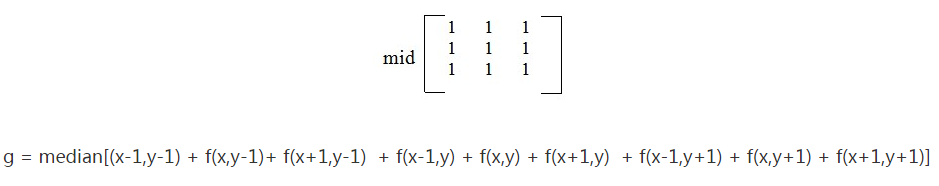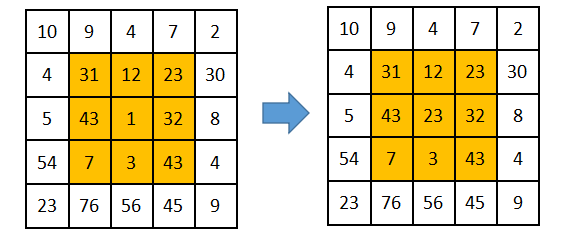代码：

//求九个数的中值
uchar Median(uchar n1, uchar n2, uchar n3, uchar n4, uchar n5,
uchar n6, uchar n7, uchar n8, uchar n9) {
uchar arr;
arr = n1;
arr = n2;
arr = n3;
arr = n4;
arr = n5;
arr = n6;
arr = n7;
arr = n8;
arr = n9;
for (int gap = 9 / 2; gap > 0; gap /= 2)//希尔排序
for (int i = gap; i < 9; ++i)
for (int j = i - gap; j >= 0 && arr[j] > arr[j + gap]; j -= gap)
swap(arr[j], arr[j + gap]);
return arr;//返回中值
}

//图像椒盐化
void salt(Mat &image, int num) {
if (!image.data) return;//防止传入空图
int i, j;
srand(time(NULL));
for (int x = 0; x < num; ++x) {
i = rand() % image.rows;
j = rand() % image.cols;
image.at<Vec3b>(i, j) = 255;
image.at<Vec3b>(i, j) = 255;
image.at<Vec3b>(i, j) = 255;
}
}

//中值滤波函数
void MedianFlitering(const Mat &src, Mat &dst) {
if (!src.data)return;
Mat _dst(src.size(), src.type());
for(int i=0;i<src.rows;++i)
for (int j=0; j < src.cols; ++j) {
if ((i - 1) > 0 && (i + 1) < src.rows && (j - 1) > 0 && (j + 1) < src.cols) {
_dst.at<Vec3b>(i, j) = Median(src.at<Vec3b>(i, j), src.at<Vec3b>(i + 1, j + 1),
src.at<Vec3b>(i + 1, j), src.at<Vec3b>(i, j + 1), src.at<Vec3b>(i + 1, j - 1),
src.at<Vec3b>(i - 1, j + 1), src.at<Vec3b>(i - 1, j), src.at<Vec3b>(i, j - 1),
src.at<Vec3b>(i - 1, j - 1));
_dst.at<Vec3b>(i, j) = Median(src.at<Vec3b>(i, j), src.at<Vec3b>(i + 1, j + 1),
src.at<Vec3b>(i + 1, j), src.at<Vec3b>(i, j + 1), src.at<Vec3b>(i + 1, j - 1),
src.at<Vec3b>(i - 1, j + 1), src.at<Vec3b>(i - 1, j), src.at<Vec3b>(i, j - 1),
src.at<Vec3b>(i - 1, j - 1));
_dst.at<Vec3b>(i, j) = Median(src.at<Vec3b>(i, j), src.at<Vec3b>(i + 1, j + 1),
src.at<Vec3b>(i + 1, j), src.at<Vec3b>(i, j + 1), src.at<Vec3b>(i + 1, j - 1),
src.at<Vec3b>(i - 1, j + 1), src.at<Vec3b>(i - 1, j), src.at<Vec3b>(i, j - 1),
src.at<Vec3b>(i - 1, j - 1));
}
else
_dst.at<Vec3b>(i, j) = src.at<Vec3b>(i, j);
}
_dst.copyTo(dst);//拷贝
}

void main() {

Mat Salt_Image;
image.copyTo(Salt_Image);
salt(Salt_Image, 3000);

Mat image3, image4;
MedianFlitering(Salt_Image, image3);
medianBlur(Salt_Image, image4, 3);
imshow("自定义中值滤波处理后", image3);
imshow("openCV自带的中值滤波", image4);
waitKey();
}


效果图：可以看到，椒盐噪声很好的被平滑了，而且也没均值那样模糊化太过于严重。

三 填充问题

在对图像应用滤波器进行过滤时，边界问题是一个需要处理的问题。一般来说，有3种处理的方法。

1. 不做边界处理

不对图像的边界作任何处理，在对图像进行滤波时，滤波器没有作用到图像的四周，因此图像的四周没有发生改变。

2. 填充0

对图像的边界做扩展，在扩展边界中填充0，对于边长为2k+1的方形滤波器，扩展的边界大小为k，若原来的图像为[m, n]，则扩展后图像变为[m+2k, n+2k]。进行滤波之后，图像会出现一条黑色的边框。

3. 填充最近像素值

扩展与 填充0 的扩展类似，只不过填充0的扩展是在扩展部分填充0，而这个方法是填充距离最近的像素的值。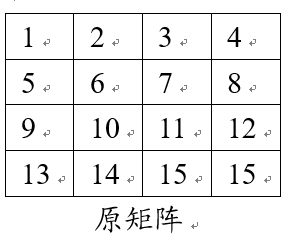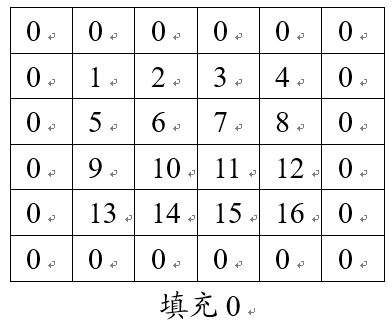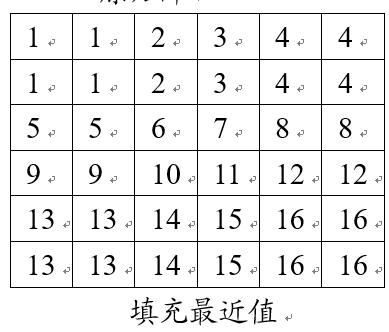四 总结：

均值滤波和和中值滤波都可以起到平滑图像，虑去噪声的功能。

均值滤波采用线性的方法，平均整个窗口范围内的像素值，均值滤波本身存在着固有的缺陷，即它不能很好地保护图像细节，在图像去噪的同时也破坏了图像的细节部分，从而使图像变得模糊，不能很好地去除噪声点。均值滤波对高斯噪声表现较好，对椒盐噪声表现较差。

中值滤波采用非线性的方法，它在平滑脉冲噪声方面非常有效,同时它可以保护图像尖锐的边缘，选择适当的点来替代污染点的值，所以处理效果好，对椒盐噪声表现较好，对高斯噪声表现较差。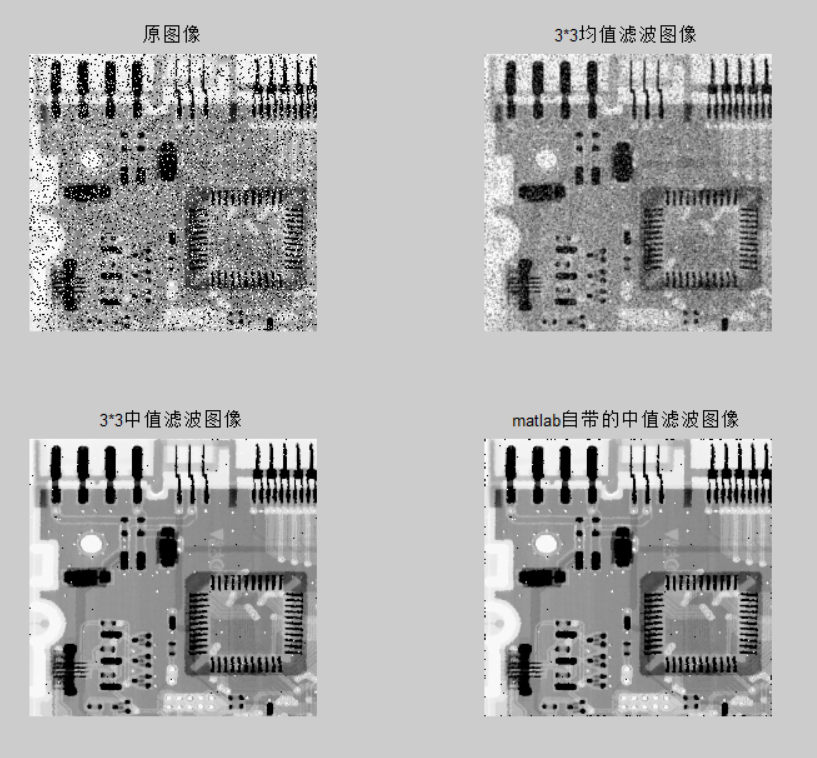参考：

1.均值滤波和中值滤波

https://blog.csdn.net/cjsh_123456/article/details/79261271

2.
展开全文图像处理 中值滤波 均值滤波
• 均值滤波均值滤波，是图像处理中最常用的手段，从频率域观点来看均值滤波是一种低通滤波器，高频信号将会去掉，因此可以帮助消除图像尖锐噪声，实现图像平滑，模糊等功能。理想的均值滤波是用每个像素和它周围像素...
均值滤波均值滤波，是图像处理中最常用的手段，从频率域观点来看均值滤波是一种低通滤波器，高频信号将会去掉，因此可以帮助消除图像尖锐噪声，实现图像平滑，模糊等功能。理想的均值滤波是用每个像素和它周围像素计算出来的平均值替换图像中每个像素。采样Kernel数据通常是3X3的矩阵，如下表示：从左到右从上到下计算图像中的每个像素，最终得到处理后的图像。均值滤波可以加上两个参数，即迭代次数，Kernel数据大小。一个相同的Kernel，但是多次迭代就会效果越来越好。同样，迭代次数相同，Kernel矩阵越大，均值滤波的效果就越明显。中值滤波中值滤波也是消除图像噪声最常见的手段之一，特别是消除椒盐噪声，中值滤波的效果要比均值滤波更好。中值滤波是跟均值滤波唯一不同是，不是用均值来替换中心每个像素，而是将周围像素和中心像素排序以后，取中值，一个3X3大小的中值滤波如下：最大最小值滤波最大最小值滤波是一种比较保守的图像处理手段，与中值滤波类似，首先要排序周围像素和中心像素值，然后将中心像素值与最小和最大像素值比较，如果比最小值小，则替换中心像素为最小值，如果中心像素比最大值大，则替换中心像素为最大值。一个Kernel矩阵为3X3的最大最小值滤波如下：双边滤波一种同时考虑了像素空间差异与强度差异的滤波器，因此具有保持图像边缘的特性。先看看高斯滤波器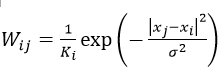其中W是权重，i和j是像素索引，K是归一化常量。公式中可以看出，权重只和像素之间的空间距离有关系，无论图像的内容是什么，都有相同的滤波效果。再来看看双边滤波器，它只是在原有高斯函数的基础上加了一项，如下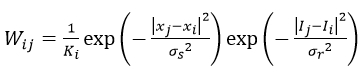其中 I 是像素的强度值，所以在强度差距大的地方（边缘），权重会减小，滤波效应也就变小。总体而言，在像素强度变换不大的区域，双边滤波有类似于高斯滤波的效果，而在图像边缘等强度梯度较大的地方，可以保持梯度。引导滤波与双边滤波最大的相似之处，就是同样具有保持边缘特性。在引导滤波的定义中，用到了局部线性模型，至于该模型，可以暂时用下图简单的理解该模型认为，某函数上一点与其邻近部分的点成线性关系，一个复杂的函数就可以用很多局部的线性函数来表示，当需要求该函数上某一点的值时，只需计算所有包含该点的线性函数的值并做平均即可。这种模型，在表示非解析函数上，非常有用。同理，我们可以认为图像是一个二维函数，而且没法写出解析表达式，因此我们假设该函数的输出与输入在一个二维窗口内满足线性关系，如下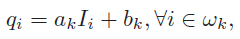其中，q是输出像素的值，I是输入图像的值，i和k是像素索引，a和b是当窗口中心位于k时该线性函数的系数。其实，输入图像不一定是待滤波的图像本身，也可以是其他图像即引导图像，这也是为何称为引导滤波的原因。对上式两边取梯度，可以得到即当输入图像I有梯度时，输出q也有类似的梯度，现在可以解释为什么引导滤波有边缘保持特性了。下一步是求出线性函数的系数，也就是线性回归，即希望拟合函数的输出值与真实值p之间的差距最小，也就是让下式最小这里p只能是待滤波图像，并不像I那样可以是其他图像。同时，a之前的系数（以后都写为e）用于防止求得的a过大，也是调节滤波器滤波效果的重要参数。通过最小二乘法，我们可以得到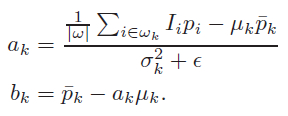其中，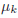是I在窗口w_k中的平均值，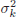是I在窗口w_k中的方差，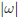是窗口w_k中像素的数量，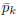是待滤波图像p在窗口w_k中的均值。在计算每个窗口的线性系数时，我们可以发现一个像素会被多个窗口包含，也就是说，每个像素都由多个线性函数所描述。因此，如之前所说，要具体求某一点的输出值时，只需将所有包含该点的线性函数值平均即可，如下这里，w_k是所有包含像素i的窗口，k是其中心位置。当把引导滤波用作边缘保持滤波器时，往往有 I = p ，如果e=0，显然a=1, b=0是E(a,b)为最小值的解，从上式可以看出，这时的滤波器没有任何作用，将输入原封不动的输出。如果e>0，在像素强度变化小的区域（或单色区域），有a近似于（或等于）0，而b近似于（或等于），即做了一个加权均值滤波；而在变化大的区域，a近似于1，b近似于0，对图像的滤波效果很弱，有助于保持边缘。而e的作用就是界定什么是变化大，什么是变化小。在窗口大小不变的情况下，随着e的增大，滤波效果越明显。在滤波效果上，引导滤波和双边滤波差不多，在一些细节上，引导滤波较好。引导滤波最大的优势在于，可以写出时间复杂度与窗口大小无关的算法，因此在使用大窗口处理图片时，其效率更高。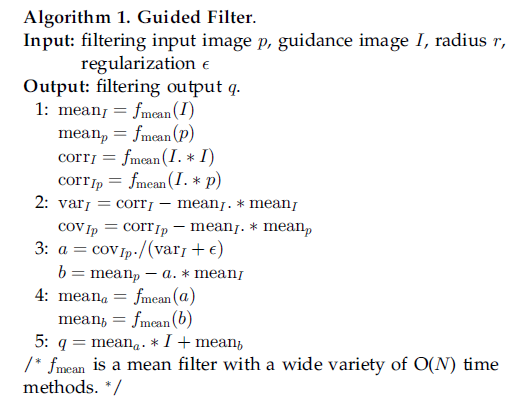[python] view plain copydef guidedfilter(I,p,r,eps):      '''''I:引导图图；     p:输入图（p=I）;     r :半径:     eps:regulation      f:为窗口半径为r的均值滤波器；     corr：相关；     var：方差；     cov：协方差     '''      height,width = I.reshape()      m_I = cv2.boxFilter(I,-1,(r,r))  #f_mean(I) 均值滤波blur和盒式滤波一样      m_p = cv2.boxFilter(p,-1,(r,r))  #f_mean(p)          m_II = cv2.boxFilter(I*I,-1,(r,r)) #f_mean(I.*I)      m_Ip = cv2.boxFilter(I * p, -1, (r, r))  #f_mean(I.*p)        var_I = m_II-m_I*m_I   #求方差：corr_I -mean_I.*mean_I      cov_Ip = m_Ip - m_I * m_p  #协方差： #cov_Ip-mean_I.*mean_p        a = cov_Ip/(var_I+eps)  #cov_Ip./(var_I+eps)      b = m_p-a*m_I   #mean_p -a.*mean_I      m_a = cv2.boxFilter(a,-1,(r,r))  #mean_a      m_b = cv2.boxFilter(b,-1,(r,r))  #mean_b      return m_a*I+m_b
展开全文• 图像中的噪声 噪声的来源：数字图像的噪声主要产生于获取、传输图像的过程。在获取图像的过程，摄像机组件的运行情况受各种客观因素的影响，包括图像拍摄的环境条件和摄像机的传感元器件质量在内都有可能会对...图像识别 iir滤波器 计算机视觉
• 一、学习心得： 在我学习基本滤波算法原理的时候，因为刚接触不是很理解算法具体是怎样实现的，不过在学习了图像形态学之后，发现滤波算法其实很简单。所以在此建议初学者在学习...而在图像处理中的滤波算法中，处...
• 图像处理 中值滤波与均值滤波 matlab算法实现
• 本文作为“保边滤波器集锦”的最后一篇，来概括一下其他的本文所未提及的保边滤波器。...而大多数频域算法，都需要将图像变换到频率域，滤波之后在转换到空间域，耗时长，优化困难，因此，本人这里未单独介绍。实...磨皮算法 保边滤波器 算法实现
• 该算法用于处理8位灰度值图像，使用N=3的值滤波的算法程序，可以很方便的转换成N=5、7、9的中值滤波算法。 经该算法处理带有椒盐噪声的图像后，可以看出与原噪声图像的不同，但是效果不是那么的明显。
• 数字图像处理中频域滤波ppt讲义，内容详尽，最后有仿真图
• 这篇文章主要讲解一下用FPGA实现图像的均值滤波算法，为什么要写这篇文章呢？ 主要原因：在图像领域里会对一些视频数据要做各种算法处理，去噪算法是用到最多的算法；当然图像去噪算法有很多种，例如均值滤波、中值...
• 数字图像处理的均值滤波，用于图像去噪。很完整的源代码均值滤波
• 对于数字图像处理，通常会用到各种的滤波操作，谈及滤波这个词，总会让人联想到信号处理中那一系列繁琐的转换计算公式，顿时觉得头昏脑胀。其实，若仅仅从空域来理解数字图像滤波操作，便能够更加直观地理解整个...导向滤波 中值滤波 双边滤波 高斯滤波
• EPM滤波即Edge preserved mean filter，算法本身来自于一篇公开专利，专利号为CN102509266：一种图像快速保边滤波方法；该专利设计了一种快速保边滤波器，可以达到双边滤波的效果，而时间复杂度为O(l)，实际上该算法...磨皮算法 保边滤波器
• 本文介绍五种常见的图像滤波方式：线性滤波（方框滤波、均值滤波、高斯滤波）；非线性滤波（中值滤波、双边滤波）。提醒，本文主要是算法公式，没有具体完整的代码。 一、线性滤波 总的来说，这三种线性滤波原理...
• 灰度图像的中值滤波算法verilog实现，并且实现了前端摄像头的采集程序。
• 图像处理之积分图应用二(快速边缘保留滤波算法) 一：基本原理 传统的图像边缘保留滤波算法-如高斯双边模糊、Mean-Shift模糊等计算复杂、效率比较低，虽然有各种手段优化或者快速计算方法，当时算法相对...
• 图像处理经典滤波算法实现，包括中值滤波、卷积、Sobel、Canny、Pyramid、Hough变换、轮廓提取、直方图均衡化、小波变换等等，这是我在国外网上下载的一个工具，可以试验算法效果，用着不错，拿来分享下。
• 局部均值滤波算法是基于均值改进的一种具有一定保边能力的滤波器，参考论文为Lee filter Digital image enhancement and noise filtering by using local statistics，具体算法如下：本人C实现效果如下：主要代码...磨皮算法
...